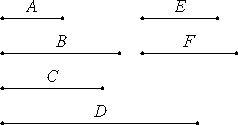# Proposition 25

If two numbers have to one another the ratio which a cubic number has to a cubic number, and the first is a cube, then the second is also a cube.

Let the two numbers A and B have to one another the ratio which the cubic number C has to the cubic number D, and let A be a cube.I say that B is also a cube.

VIII.19

Since C and D are cubes, C and D are similar solid numbers, therefore two mean proportional numbers fall between C and D.

VIII.18

Since as many numbers fall in continued proportion between those which have the same ratio with C and D as fall between C and D, therefore two mean proportional numbers E and F fall between A and B.

VIII.23

Since, then, the four numbers A, E, F, and B are in continued proportion, and A is a cube, therefore B is also a cube.

Therefore, if two numbers have to one another the ratio which a cubic number has to a cubic number, and the first is a cube, then the second is also a cube.

Q.E.D.

## Guide

This proposition is analogous to the previous one about squares. Its proof is straightforward.

This proposition is used in IX.10.